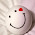## Tuesday, 26 January 2016

### 9 Things About GRE Maths That Will Help You Score 165+

Each of the two scored Quantitative sections consists of 20 questions in 35 minutes. Also, GRE is a section-level adaptive exam, meaning that your performance on your first math section will determine the difficulty of the next math section you will receive. Since there is no penalty for wrong answers in GRE, you can always try guessing in some questions, it’s always better than leaving it unanswered.

Following is the Plan of Action that a GRE aspirant should implant into his/her preparation to ace the Quant section of GRE exam:

#### 1. RTFQ - Read The Full Question

Try to thoroughly analyze each & every information given in the question as every information is important & leads us to the answer.

#### 2. Use the Options

Sometimes it’s much better to solve the questions from options. If it is possible, try to do the question from options than to do it the longer way. Always remember that numeric options in GRE are either in ascending or descending order.

#### 3. Equations driven vs Formula driven problems

Formula driven problems generally don’t take much time, but be very careful with Equations driven problems, which can be tricky at times. Please divide the question into small pieces and then solve each piece to reach final answer. Allot more time on word problems.

#### 4. POOD - Personal order of difficulty

Never attempt a question on predefined assumptions. E.g. "probability questions are not always hard".

#### 5. Break Complex figures to smaller shapes

Look for known figures and formulae while solving complex geometry questions.

#### 6. Analyze tables/graphs thoroughly

It is not necessary not use everything given. Read the question and decide which chunk is required to answer the question.

#### 7. Time allotted per Question

On an average, you get around 1 minute 45 seconds to solve every question. Try to complete the easy questions as soon as possible and then solve the difficult questions in the remaining time.

#### 8. Use of Calculator

Avoid calculator for simple calculations and try doing them in your mind. This would save time.

#### 9. Tough areas for GRE

Areas that might require more practice are: -
• Permutations and Combination
• Standard Deviation
• Probability
• Remainders
• Sets: Triple Venn Diagrams
• Time and Work

Learn all the concepts in detail to score a 165+. Some students would skip some topics assuming that only 1-2 questions will come from those topics, but often it turns out that even 5-6 questions are asked and getting a 165+ becomes very difficult.

In all target around 300-400 tough questions during your Quant prep which will require around 15-25 hours of preparation.

For best practice questions and mock tests visit www.wiseswan.com

#### 1 comment:

1.Thanks for sharing the Tips.. Yeah. One can get the Aspired score by Practicing the Quant as per GRE Quant Syllabus.